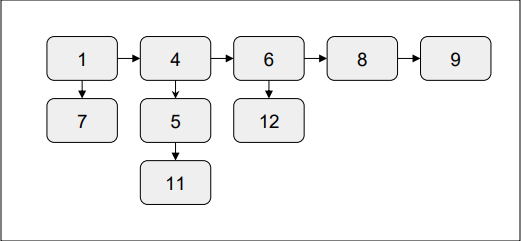# Flattening a Linked list in C++

In this problem, we are given linked list consisting of two pointer nodes, right and down.

• Right node is the main linked list pointer.

• Down node is for secondary linked list starting with that node.

All the linked lists are sorted.

Our task is to create a program to flatten a linked list and the resulting list will itself be a sorted one.

Let’s take an example to understand the problem

InputOutput

1-> 9-> 8 -> 4 -> 6-> 7-> 2-> 3-> 5

## Solution Approach

A solution to the problem is using merge sort for a linked list. This method will merge the lists recursively in a sorted order to form a flattened list.

## Example

Program to illustrate the working of our solution

#include <bits/stdc++.h>
using namespace std;

class Node{
public:
int data;
Node *right, *down;
};
Node* mergeList(Node* a, Node* b){
if (a == NULL)
return b;
if (b == NULL)
return a;
Node* result;
if (a->data < b->data){
result = a;
result->down = mergeList(a->down, b);
}
else{
result = b;
result->down = mergeList(a, b->down);
}
result->right = NULL;
return result;
}
if (root == NULL || root->right == NULL)
return root;
root = mergeList(root, root->right);
return root;
}
Node* new_node = new Node();
new_node->data = data;
new_node->right = NULL;
}
int main(){
cout<<"The Flattened Linked list is : \n";
while (temp != NULL){
cout<<temp->data<<" => ";
temp = temp->down;
}
cout<<"NULL";
return 0;
}

## Output

The Flattened Linked list is :
1 => 4 => 5 => 6 => 7 => 8 => 11 => 12 => 16 => NULL

Updated on: 01-Feb-2022

241 Views